# 第四次随笔作业

https://www.cnblogs.com/ranh941/p/7999266.html

## 7-2 求最大值及其下标

#include<stdio.h>
int main()
{
int n=0,i=0,max=0,m=0;
scanf("%d",&n);
int a[n];
for(i=0;i<n;i++)
{
scanf("%d",&a[i]);
}
max=a;
for(i=0;i<n;i++)
{
if(max<a[i]){
max=a[i];
m=i;
}
}
printf("%d %d",max,m);
return 0;
} 

第一步：定义四个整形变量n，i，max，m并将其初始化，输入n，并定义拥有n个数字的数组a。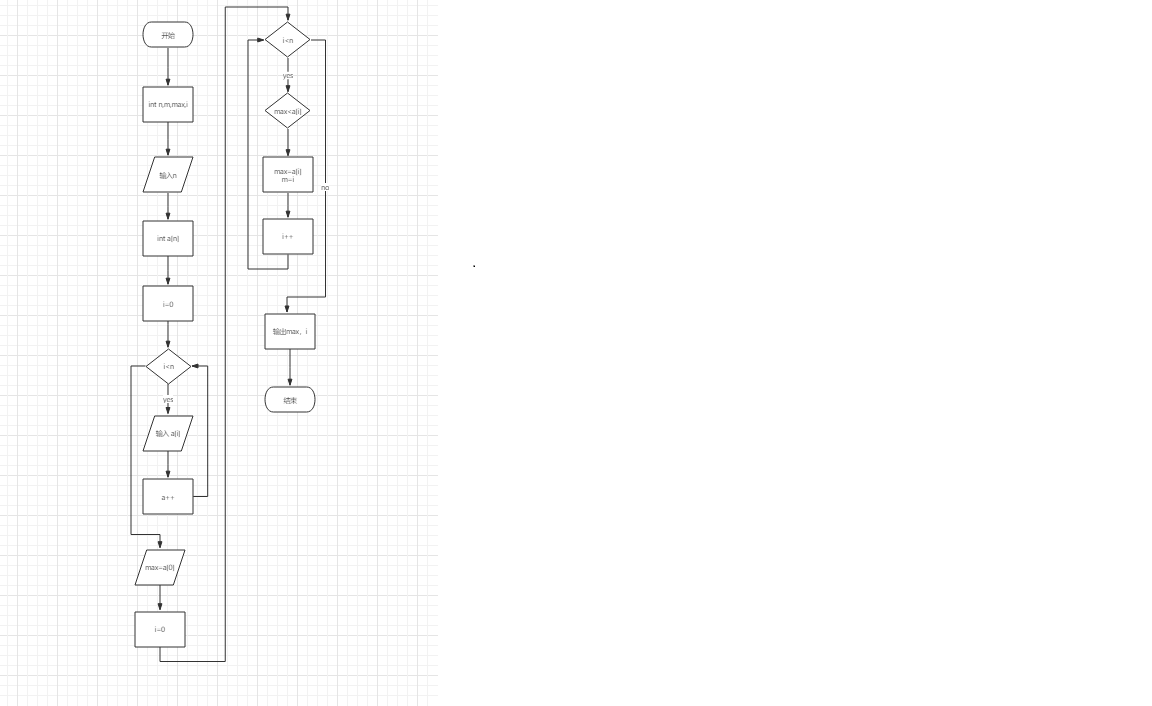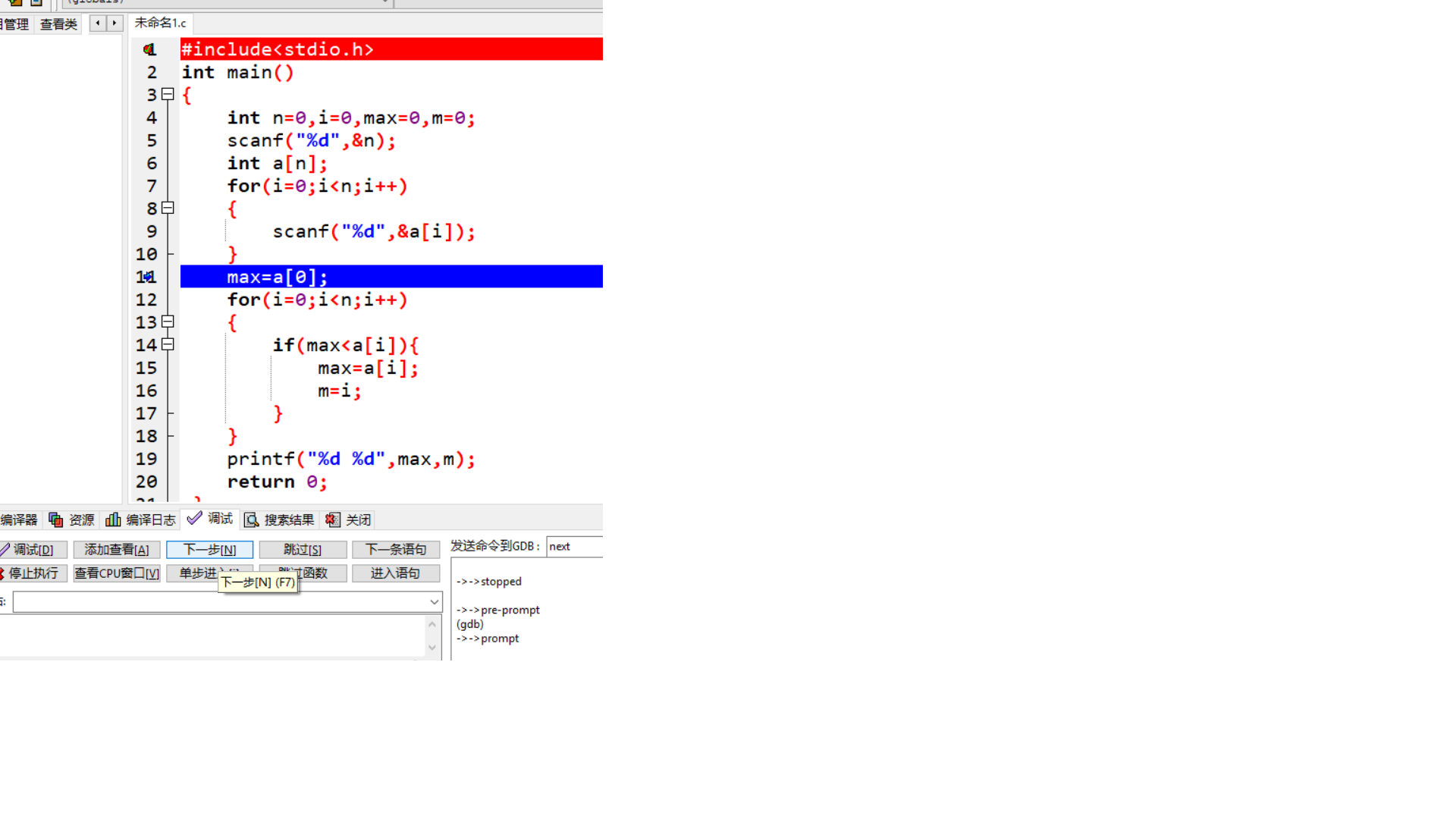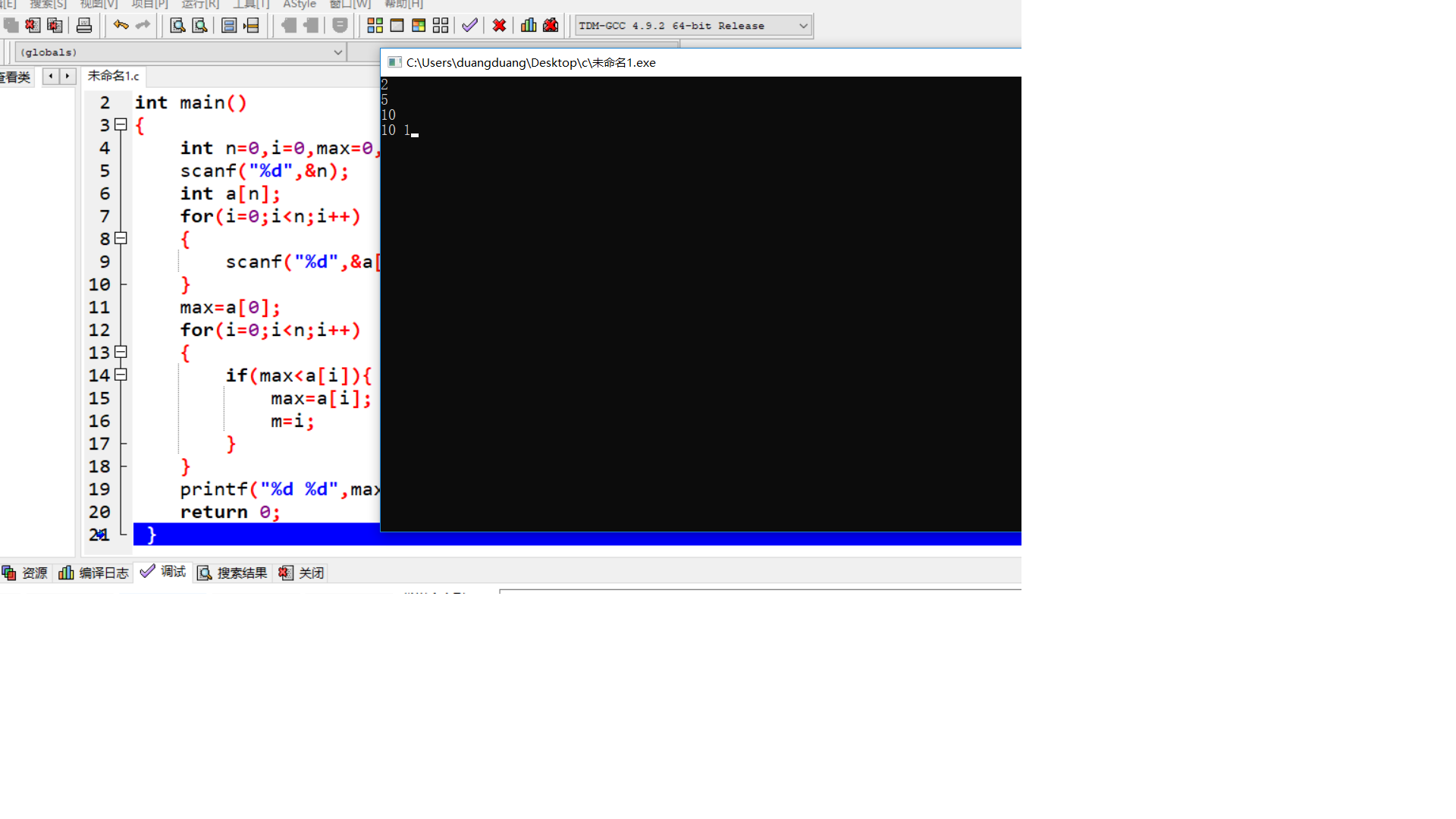## 7-3 交换最小值和最大值

#include<stdio.h>
int main()
{
int n,m=0,i=0,max=0,min=0,t=0,c=0,d=0;
scanf("%d",&n);
int a[n];
for(i=0;i<n;i++)
{
scanf("%d",&a[i]);
}
max=a;
min=a;
for(i=0;i<n;i++)
{
if(a[i]>max)
{
max=a[i];
c=i;
}
if(a[i]<min)
{
min=a[i];
d=i;
}
}
m=a;
t=a[d];
a[d]=a;
a=t;
if(c!=0){
t=a[c];
a[c]=a[n-1];
a[n-1]=t;
}
else{
t=a[n-1];
a[n-1]=m;
a[d]=t;
}
for(i=0;i<n;i++)
{
printf("%d ",a[i]);
}
return 0;
}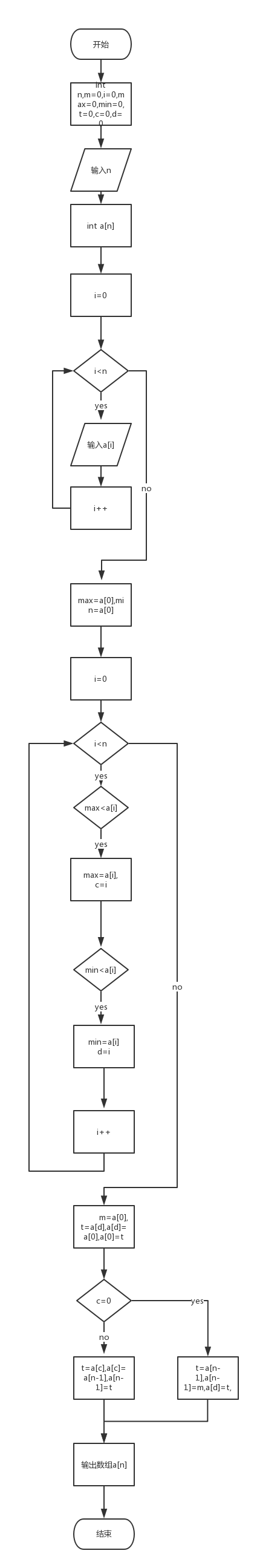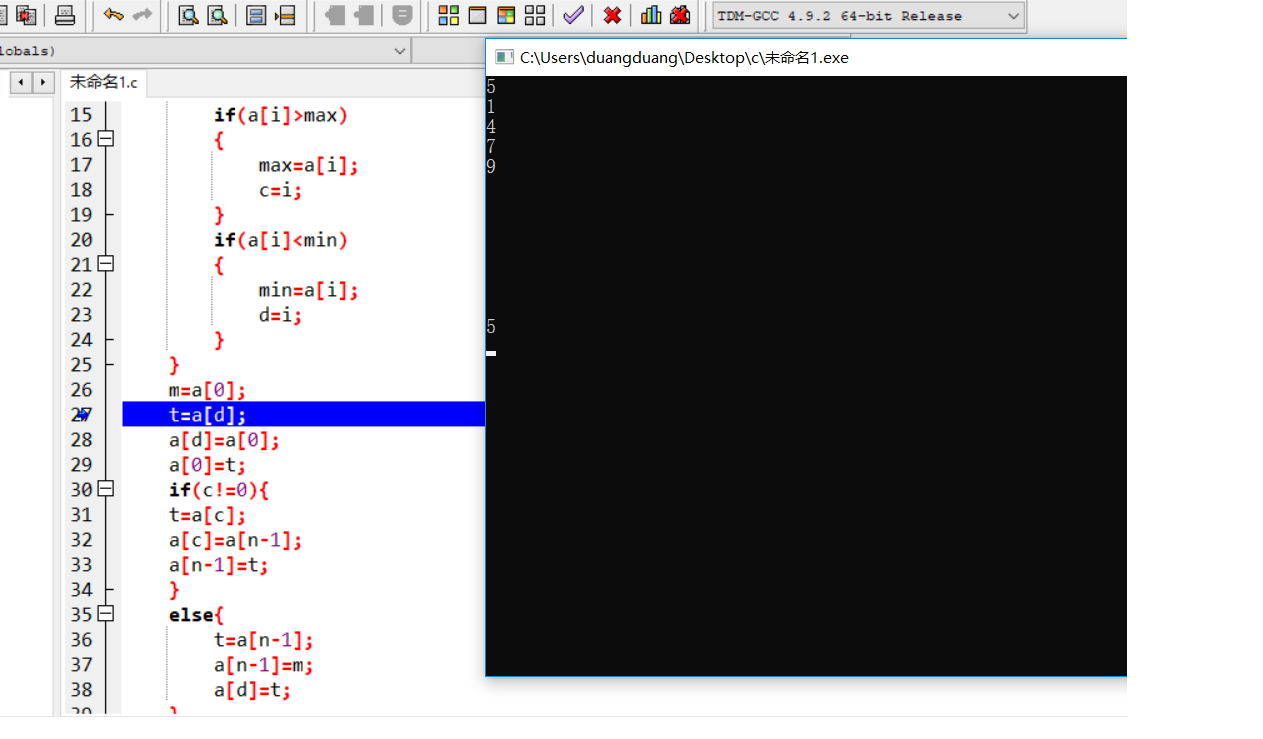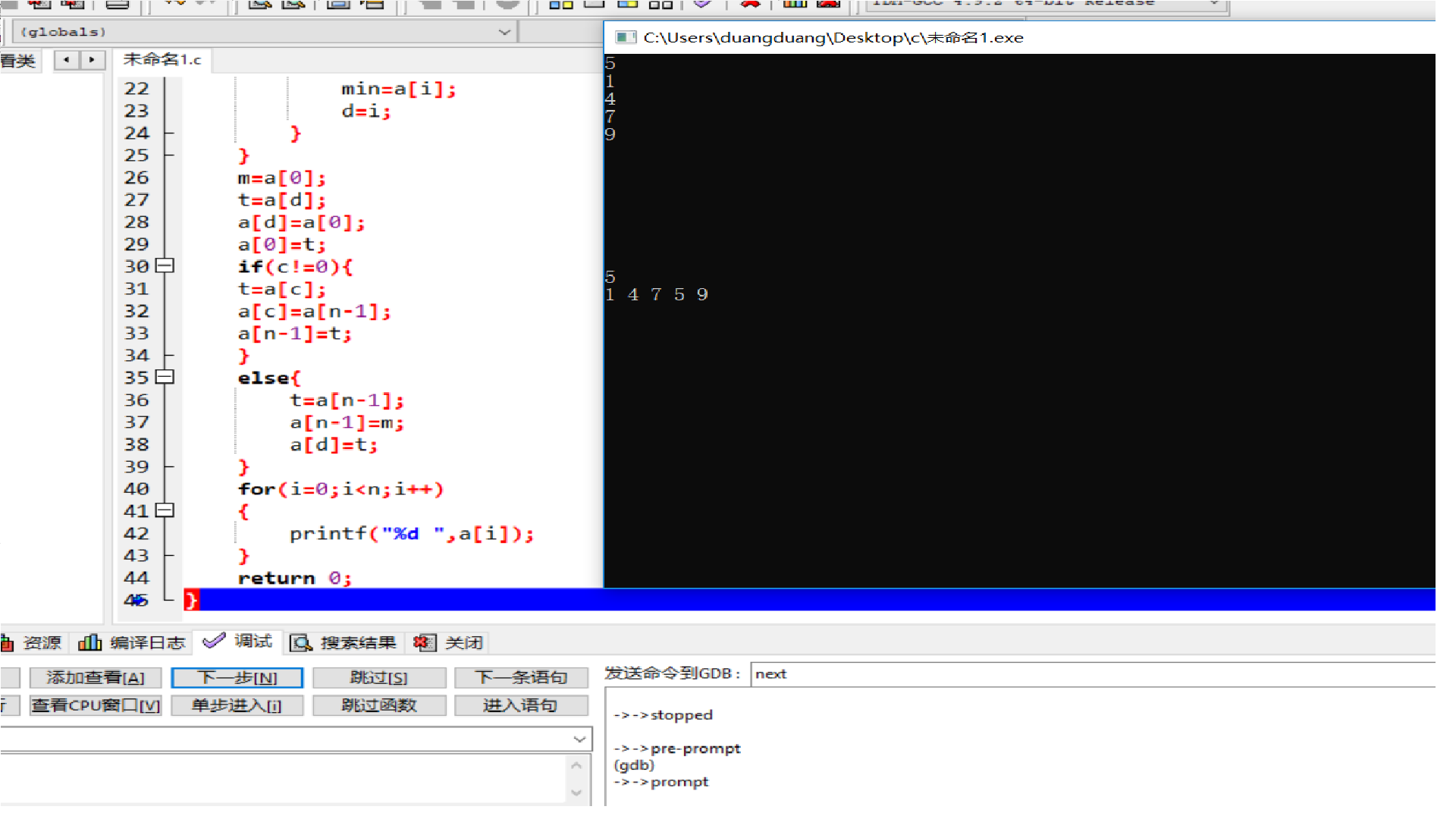## 统计图表：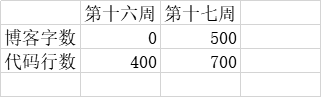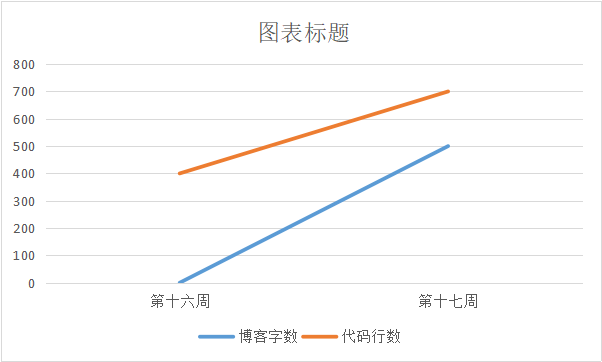https://www.cnblogs.com/159357www/p/10165685.html

https://www.cnblogs.com/chengxu230921/p/10170865.html

posted @ 2018-12-25 18:36  张洸溥。  阅读(155)  评论(6编辑  收藏  举报